Skip to main content Accessibility help
Home
Hostname: page-component-78dcdb465f-mrc2z Total loading time: 12.31 Render date: 2021-04-16T11:37:37.707Z Has data issue: true Feature Flags: { "shouldUseShareProductTool": true, "shouldUseHypothesis": true, "isUnsiloEnabled": true, "metricsAbstractViews": false, "figures": false, "newCiteModal": false, "newCitedByModal": true }Compositio Mathematica

# Classification of universal formality maps for quantizations of Lie bialgebras

Published online by Cambridge University Press:  27 November 2020

Corresponding
E-mail address:

## Abstract

We settle several fundamental questions about the theory of universal deformation quantization of Lie bialgebras by giving their complete classification up to homotopy equivalence. Moreover, we settle these questions in a greater generality: we give a complete classification of the associated universal formality maps. An important new technical ingredient introduced in this paper is a polydifferential endofunctor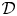${\mathcal {D}}$ in the category of augmented props with the property that for any representation of a prop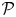${\mathcal {P}}$ in a vector space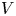$V$ the associated prop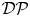${\mathcal {D}}{\mathcal {P}}$ admits an induced representation on the graded commutative algebra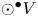$\odot ^\bullet V$ given in terms of polydifferential operators. Applying this functor to the minimal resolution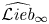$\widehat {\mathcal {L}\textit{ieb}}_\infty$ of the genus completed prop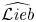$\widehat {\mathcal {L}\textit{ieb}}$ of Lie bialgebras we show that universal formality maps for quantizations of Lie bialgebras are in one-to-one correspondence with morphisms of dg props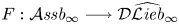$F: \mathcal{A}\textit{ssb}_\infty \longrightarrow {\mathcal{D}}\widehat{\mathcal{L}\textit{ieb}}_\infty$
satisfying certain boundary conditions, where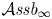$\mathcal {A}\textit{ssb}_\infty$ is a minimal resolution of the prop of associative bialgebras. We prove that the set of such formality morphisms is non-empty. The latter result is used in turn to give a short proof of the formality theorem for universal quantizations of arbitrary Lie bialgebras which says that for any Drinfeld associator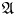$\mathfrak{A}$ there is an associated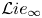${\mathcal {L}} ie_\infty$ quasi-isomorphism between the${\mathcal {L}} ie_\infty$ algebras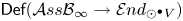$\mathsf {Def}({\mathcal {A}} ss{\mathcal {B}}_\infty \rightarrow {\mathcal {E}} nd_{\odot ^\bullet V})$ and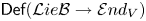$\mathsf {Def}({\mathcal {L}} ie{\mathcal {B}}\rightarrow {\mathcal {E}} nd_V)$ controlling, respectively, deformations of the standard bialgebra structure in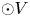$\odot V$ and deformations of any given Lie bialgebra structure in$V$. We study the deformation complex of an arbitrary universal formality morphism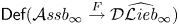$\mathsf {Def}(\mathcal {A}\textit{ssb}_\infty \stackrel {F}{\rightarrow } {\mathcal {D}}\widehat {\mathcal {L}\textit{ieb}}_\infty )$ and prove that it is quasi-isomorphic to the full (i.e. not necessary connected) version of the graph complex introduced Maxim Kontsevich in the context of the theory of deformation quantizations of Poisson manifolds. This result gives a complete classification of the set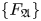$\{F_\mathfrak{A}\}$ of gauge equivalence classes of universal Lie connected formality maps: it is a torsor over the Grothendieck–Teichmüller group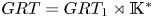$GRT=GRT_1\rtimes {\mathbb {K}}^*$ and can hence can be identified with the set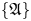$\{\mathfrak{A}\}$ of Drinfeld associators.

## MSC classification

Type
Research Article
Information
Compositio Mathematica , October 2020 , pp. 2111 - 2148
Copyright
© The Author(s) 2020

## Access options

Get access to the full version of this content by using one of the access options below.

## Footnotes

S.M. has been partially supported by the Swedish Vetenskaprådet, grant 2012-5478. T.W. has been partially supported by the Swiss National Science foundation, grant 200021_150012, and the SwissMAP NCCR funded by the Swiss National Science foundation.

## References

Alm, J., Two-colored noncommmutative Gerstenhaber formality and infinity Duflo isomorphism, Preprint (2011), arXiv:1104.2194.Google Scholar
Drinfeld, V., On quasitriangular quasi-Hopf algebras and a group closely connected with$Gal(\bar {\mathbb {Q}}/\mathbb {Q})$, Leningrad Math. J. 2 (1991), 829860.Google Scholar
Drinfeld, V., On some unsolved problems in quantum group theory, Lecture Notes in Mathematics, vol. 1510 (Springer, 1992), 18.Google Scholar
Enriquez, B., Quantization of Lie bialgebras and shuffle algebras of Lie algebras, Adv. Math. 164 (2001), 123.CrossRefGoogle Scholar
Enriquez, B., On some universal algebras associated to the category of Lie bialgebras, Selecta Math. (N.S.) 7 (2001), 321407.CrossRefGoogle Scholar
Enriquez, B. and Etingof, P., On the invertability of quantization functors, Adv. Math. 197 (2005), 430479.CrossRefGoogle Scholar
Etingof, P. and Kazhdan, D., Quantization of Lie bialgebras, I, Selecta Math. (N.S.) 2 (1996), 141.CrossRefGoogle Scholar
Gerstenhaber, M. and Schack, S. D., Bialgebra cohomology, deformations, and quantum groups, Proc. Natl. Acad. Sci. USA 87 (1990), 478481.CrossRefGoogle ScholarPubMed
Hoefel, E. and Livernet, M., Open-closed homotopy algebras and strong homotopy Leibniz pairs through Koszul Operad Theory, Lett. Math. Phys. 101 (2012), 195222.CrossRefGoogle Scholar
Kapranov, M. and Manin, Yu. I., Modules and Morita theorem for operads, Amer. J. Math. 123 (2001), 811838.CrossRefGoogle Scholar
Kontsevich, M., Formality conjecture, in Deformation theory and symplectic geometry, eds D. Sternheimer et al. (Kluwer, 1997), 139–156.Google Scholar
Kontsevich, M., Deformation quantization of Poisson manifolds, Lett. Math. Phys. 66 (2003), 157216.CrossRefGoogle Scholar
Markl, M., A resolution (minimal model) of the prop for bialgebras, J. Pure Appl. Algebra 205 (2006), 341374.CrossRefGoogle Scholar
Markl, M. and Voronov, A. A., PROPped-up graph cohomology, Algebra, arithmetic, and geometry: in honor of Yu. I. Manin, volume II, Progress in Mathematics, vol. 270 (Birkhaüser, Boston, MA, 2009), 249281.CrossRefGoogle Scholar
Merkulov, S. A., Permutahedra, HKR isomorphism and polydifferential Gerstenhaber-Schack complex, Higher structure in geometry and physics: in honor of Murray Gerstenhaber and Jim Stasheff, Progress in Mathematics, vol. 287 (Birkhaüser, Boston, MA, 2011), 293314.CrossRefGoogle Scholar
Merkulov, S. A., Formality theorem for quantizations of Lie bialgebras, Lett. Math. Phys. 106 (2016), 169195.CrossRefGoogle Scholar
Merkulov, S. and Vallette, B., Deformation theory of representations of prop(erad)s I & II, J. Reine Angew. Math. 634 (2009), 51106, 636 (2009), 123–174.Google Scholar
Merkulov, S. and Willwacher, T., Props of ribbon graphs, involutive Lie bialgebras and moduli spaces of curves, Preprint (2015), arXiv:1511.07808.Google Scholar
Merkulov, S. and Willwacher, T., Deformation theory of Lie bialgebra properads, in Geometry and physics: a Festschrift in honour of Nigel Hitchin (Oxford University Press, 2018), 219–248.CrossRefGoogle Scholar
Merkulov, S. and Willwacher, T., An explicit two step quantization of Poisson structures and Lie bialgebras, Comm. Math. Phys. 364 (2018), 505578.CrossRefGoogle Scholar
Ševera, P., Quantization of Lie bialgebras revisited, Selecta Math. (N.S.) 22 (2016), 15631581.CrossRefGoogle Scholar
Shoikhet, B., An explicit formula for the deformation quantization of Lie bialgebras, Preprint (2004), arXiv:math.QA/0402046.Google Scholar
Tamarkin, D. E., Quantization of Lie bialgebras via the formality of the operad of little disks, Geom. Funct. Anal. 17 (2007), 537604.CrossRefGoogle Scholar
Thurston, D. P., Integral expressions for the Vassiliev knot invariants, Preprint (1999), arXiv:math.QA/9901110.Google Scholar
Vallette, B., A Koszul duality for props, Trans. Amer. Math. Soc. 359 (2007), 48654943.CrossRefGoogle Scholar
Willwacher, T., M. Kontsevich's graph complex and the Grothendieck-Teichmueller Lie algebra, Invent. Math. 200 (2015), 671760.CrossRefGoogle Scholar
Willwacher, T., Oriented graph complexes, Comm. Math. Phys. 334 (2015), 16491666.CrossRefGoogle Scholar
Willwacher, T., Models for the$n$-Swiss Cheese operads, Preprint (2015), arXiv:1506.07021.Google Scholar

### Full text views

Full text views reflects PDF downloads, PDFs sent to Google Drive, Dropbox and Kindle and HTML full text views.

Total number of HTML views: 18
Total number of PDF views: 22 *
View data table for this chart

* Views captured on Cambridge Core between 27th November 2020 - 16th April 2021. This data will be updated every 24 hours.

# Send article to Kindle

To send this article to your Kindle, first ensure no-reply@cambridge.org is added to your Approved Personal Document E-mail List under your Personal Document Settings on the Manage Your Content and Devices page of your Amazon account. Then enter the ‘name’ part of your Kindle email address below. Find out more about sending to your Kindle. Find out more about sending to your Kindle.

Note you can select to send to either the @free.kindle.com or @kindle.com variations. ‘@free.kindle.com’ emails are free but can only be sent to your device when it is connected to wi-fi. ‘@kindle.com’ emails can be delivered even when you are not connected to wi-fi, but note that service fees apply.

Find out more about the Kindle Personal Document Service.

Classification of universal formality maps for quantizations of Lie bialgebras
Available formats
×

# Send article to Dropbox

To send this article to your Dropbox account, please select one or more formats and confirm that you agree to abide by our usage policies. If this is the first time you use this feature, you will be asked to authorise Cambridge Core to connect with your <service> account. Find out more about sending content to Dropbox.

Classification of universal formality maps for quantizations of Lie bialgebras
Available formats
×

# Send article to Google Drive

To send this article to your Google Drive account, please select one or more formats and confirm that you agree to abide by our usage policies. If this is the first time you use this feature, you will be asked to authorise Cambridge Core to connect with your <service> account. Find out more about sending content to Google Drive.

Classification of universal formality maps for quantizations of Lie bialgebras
Available formats
×
×

#### Conflicting interests

Do you have any conflicting interests? *